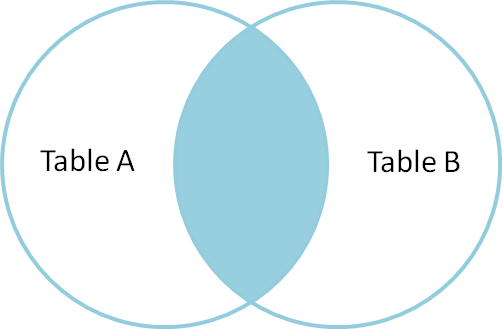# Parallel PostGIS Joins

In my earlier post on new parallel query support coming in PostgreSQL 9.6 I was unable to come up with a parallel join query, despite much kicking and thumping the configuration and query.It turns out, I didn’t have all the components of my query marked as `PARALLEL SAFE`, which is required for the planner to attempt a parallel plan. My query was this:

And `_ST_Intersects()` was marked as safe, but I neglected to mark the function behind the `&&` operator – `geometry_overlaps` – as safe. With both functions marked as safe, and assigned a hefty function cost of 1000, I get this query:

`````` Nested Loop
(cost=0.28..1264886.46 rows=21097041 width=2552)
(actual time=0.119..13876.668 rows=69534 loops=1)
->  Seq Scan on pd
(cost=0.00..14271.34 rows=69534 width=2512)
(actual time=0.018..89.653 rows=69534 loops=1)
->  Index Scan using pts_gix on pts
(cost=0.28..17.97 rows=2 width=40)
(actual time=0.147..0.190 rows=1 loops=69534)
Index Cond: (pd.geom && geom)
Filter: _st_intersects(pd.geom, geom)
Rows Removed by Filter: 2
Planning time: 8.365 ms
Execution time: 13885.837 ms
``````

Hey wait! That’s not parallel either!

It turns out that parallel query involves a secret configuration sauce, just like parallel sequence scan and parellel aggregate, and naturally it’s different from the other modes (gah!)

The default `parallel_tuple_cost` is 0.1. If we reduce that by an order of magnitude, to 0.01, we get this plan instead:

`````` Gather
(cost=1000.28..629194.94 rows=21097041 width=2552)
(actual time=0.950..6931.224 rows=69534 loops=1)
Number of Workers: 3
->  Nested Loop
(cost=0.28..628194.94 rows=21097041 width=2552)
(actual time=0.303..6675.184 rows=17384 loops=4)
->  Parallel Seq Scan on pd
(cost=0.00..13800.30 rows=22430 width=2512)
(actual time=0.045..46.769 rows=17384 loops=4)
->  Index Scan using pts_gix on pts
(cost=0.28..17.97 rows=2 width=40)
(actual time=0.285..0.366 rows=1 loops=69534)
Index Cond: (pd.geom && geom)
Filter: _st_intersects(pd.geom, geom)
Rows Removed by Filter: 2
Planning time: 8.469 ms
Execution time: 6945.400 ms
``````

Ta da! A parallel plan, and executing almost twice as fast, just like the doctor ordered.

### Complaints

Mostly the parallel support in core “just works” as advertised. PostGIS does need to mark our functions as quite costly, but that’s reasonable since they actually are quite costly. What is not good is the need to tweak the configuration once the functions are properly costed:

• Having to cut `parallel_tuple_cost` by a factor of 10 for the join case is not any good. No amount of `COST` increases seemed to have an effect, only changing the core parameter did.
• Having to increase the cost of functions used in aggregates by a factor of 100 over cost of functions used in sequence filters is also not any good.

So, with a few more changes to PostGIS, we are quite close, but the planner for parallel cases needs to make more rational use of function costs before we have achieved parallel processing nirvana for PostGIS.